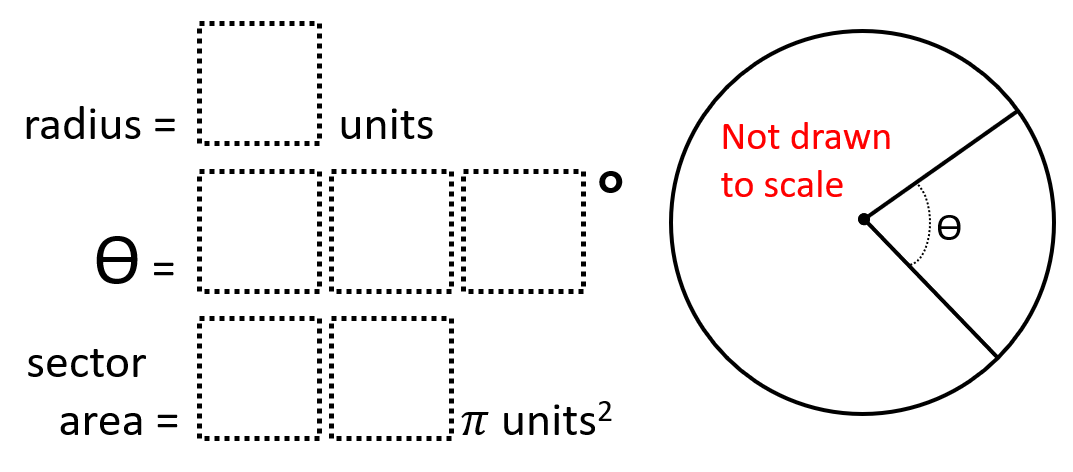# Sector Area 1

Directions: Using the digits 0 to 9 at most one time each, place a digit in each box so that the radius and angle measure result in the sector area.### Hint

How can you determine which radii or angle measures would be unrealistic possibilities without calculating them?

theta = 360°
sector area = 49 units2

theta = 135°
sector area = 24 units2

theta = 280°
sector area = 63 units2